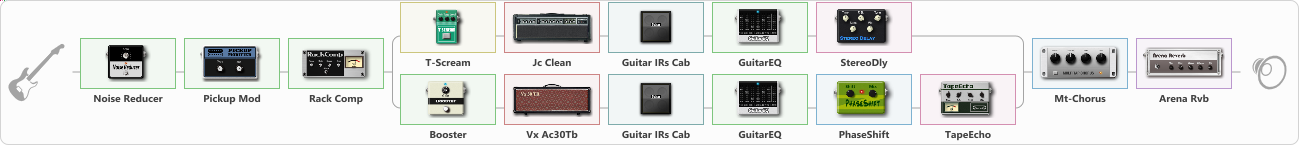# Temple Of The King_Clean

Discussion in 'ToneLib-GFX presets' started by d4nte1988, Oct 6, 2022.

1. Temple Of The King_Clean

Preset name: Temple Of The King_Clean

Clean

Effects chain:Effect: "Noise Reducer" (Dynamics / Filter), active - "yes"
{
"Sens" = 63
"Mode" = Soft
}

Effect: "Pickup Mod" (Dynamics / Filter), active - "yes"
{
"Mode" = Humbacker->Single
"Tone" = 66
}

Effect: "Rack Comp" (Dynamics / Filter), active - "yes"
{
"Threshold (dB)" = 4
"Ratio" = 17
"Attack" = Fast
"Release (ms)" = 543
"Knee" = 28
"Level (dB)" = 1
}

Effect: "Splitter" (Dynamics / Filter)
{
"A-Bypass" = Off
"A-Pan" = -26
"A-Level" = 76
"B-Bypass" = Off
"B-Pan" = 51
"B-Level" = 53
"Width" = 0

'A' branch:
{

Effect: "T-Scream" (Овердрайв / Дисторшн), active - "yes"
{
"Drive" = 32
"Tone" = 66
"Level" = 70
}

Effect: "Jc Clean" (Симуляторы усилителей), active - "yes"
{
"Gain" = 73
"Bass" = 44
"Middle" = 65
"Treble" = 66
"Presence" = 78
"Master" = 69
"Level (dB)" = 0
}

Effect: "Guitar IRs Cab" (Кабинеты), active - "yes"
{
"Model" = Marshall 1960b (4x12")
"Mic Position" = Middle
"Mic Distance" = Middle
"Low Cut (Hz)" = 0
"Hi Cut (kHz)" = 20.0
"Mix" = 100
"Level (dB)" = 4
}

Effect: "GuitarEQ" (Dynamics / Filter), active - "yes"
{
"160 Hz" = 3
"400 Hz" = 3
"800 Hz" = 2
"1.6 kHz" = -7
"3.2 kHz" = -2
"6.4 kHz" = -9
"12 kHz" = -15
"Level (dB)" = 0
}

Effect: "StereoDly" (Дилей), active - "yes"
{
"Time" = 689
"Feedback" = 41
"Tone" = 76
"Sens" = 75
"Mix" = 68
}
}
'B' branch:
{

Effect: "Booster" (Dynamics / Filter), active - "yes"
{
"Gain" = 73
}

Effect: "Vx Ac30Tb" (Симуляторы усилителей), active - "yes"
{
"Gain" = 62
"Bass" = 57
"Middle" = 100
"Treble" = 20
"Presence" = 50
"Master" = 68
"Level (dB)" = 0
}

Effect: "Guitar IRs Cab" (Кабинеты), active - "yes"
{
"Model" = Marshall JCM2000 (4x12")
"Mic Position" = Middle
"Mic Distance" = Middle
"Low Cut (Hz)" = 250
"Hi Cut (kHz)" = 20.0
"Mix" = 100
"Level (dB)" = 4
}

Effect: "GuitarEQ" (Dynamics / Filter), active - "yes"
{
"160 Hz" = -7
"400 Hz" = -5
"800 Hz" = -1
"1.6 kHz" = -7
"3.2 kHz" = 8
"6.4 kHz" = -8
"12 kHz" = -13
"Level (dB)" = 0
}

Effect: "PhaseShift" (Модуляция / Sfx), active - "yes"
{
"Shift" = 85
"Mix" = 86
}

Effect: "TapeEcho" (Дилей), active - "yes"
{
"Time" = 506
"Feedback" = 42
"Tone" = 45
"LoDamp" = 60
"Mix" = 65
}
}
}

Effect: "Mt-Chorus" (Модуляция / Sfx), active - "yes"
{
"Speed" = 5.7
"Depth" = 42
"Time" = 2.5
"Mix" = 23
}

Effect: "Arena Rvb" (Реверберация), active - "yes"
{
"Time" = 8.0
"PreDelay" = 37
"LoDamp" = 29
"HiDamp" = 35
"Mix" = 60
}

Note: You will need to download and install the ToneLib-GFX software to use the preset.

#### Attached Files:

• ###### Temple_Of_The_KingClean.tlgfx
File size:
1.5 KB
Views:
2,376
Pierre8310 and Jack McCracken like this.